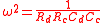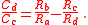xWien bridgeEncyclopedia
The Wien bridge is a type of bridge circuit
Bridge circuit
A bridge circuit is a type of electrical circuit in which two circuit branches are "bridged" by a third branch connected between the first two branches at some intermediate point along them. The bridge was originally developed for laboratory measurement purposes and one of the intermediate...

that was developed by Max Wien
Max Wien
Max Wien was a German physicist and the director of the Institute of Physics at the University of Jena. He was born in Königsberg, Prussia.Wien studied under Helmholtz and Kundt. He invented the "Löschfunkensender" during the years 1906 to 1909 and the Wien bridge in 1891...

in 1891. The bridge comprises four resistor
Resistor
A linear resistor is a linear, passive two-terminal electrical component that implements electrical resistance as a circuit element.The current through a resistor is in direct proportion to the voltage across the resistor's terminals. Thus, the ratio of the voltage applied across a resistor's...

s and two capacitor
Capacitor
A capacitor is a passive two-terminal electrical component used to store energy in an electric field. The forms of practical capacitors vary widely, but all contain at least two electrical conductors separated by a dielectric ; for example, one common construction consists of metal foils separated...

s.

Bridge circuits were a common way of measuring component values by comparing them to known values. Often an unknown component would be put in one arm of a bridge, and then the bridge would be nulled by adjusting the other arms or changing the frequency of the voltage source. See, for example, the Wheatstone bridge
Wheatstone bridge
A Wheatstone bridge is an electrical circuit used to measure an unknown electrical resistance by balancing two legs of a bridge circuit, one leg of which includes the unknown component. Its operation is similar to the original potentiometer. It was invented by Samuel Hunter Christie in 1833 and...

.

The Wien bridge is one of many common bridges. Wien's bridge is used for precison measurement of capacitance in terms of resistance and frequency. It was also used to measure audio frequencies.

The Wien bridge does not require equal values of R or C. At some frequency, the reactance of the series Rc–Cc arm will be an exact multiple of the shunt Rd–Cd arm. If the two Ra and Rb arms are adjusted to the same ratio, then the bridge is balanced.

The bridge is balanced when:andThe equations simplify if one chooses Rc = Rd and Cc = Cd; the result is Rb = 2 Ra.

In practice, the values of R and C will never be exactly equal, but the equations above show that for fixed values in the c and d arms, the bridge will balance at some ω and some ratio of Rb/Ra.